Home | Gadget | Fuzzy | Lecturer  | Power  | Transceiver

Find more type here...

Circuit explanation for +30V power supply
Seiichi Inoue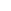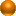The oscillation circuit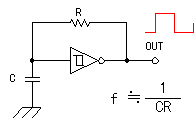The inverter which has the hysteresis characteristic is used as the oscillation circuit. The output wave form of the oscillator is the square wave. The oscillation frequency can be changed by VR1. The control of the oscillation frequency is used for the adjustment of the output voltage. The circuit which was made this time could be changed from 334KHz to 12,650KHz. The frequency with the upper limit depends on the high frequency characteristic of the IC. As for the operation of the oscillator which used the inverter, refer to "Square wave oscillator".The current amplification circuit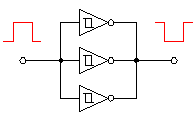It depends on the kind of the IC but the many electric currents which are gotten from the one inverter don't occur. In case of 74HC14, the maximum electric current which can be passed with the inverter of the 1 circuit is 4 mA. Because it is, the one inverter is OK but connects the inverter in parallel for the safety. In case of 74HC14, the six inverters are housed in the one package. At the circuit this time, the four inverters are connected in parallel. The inverter immediately behind the inverter for the oscillation is for the buffer. It is the one to make that the change of the load current influences the oscillation little. I think that it is permitted to do the five inverters in the multiple connection without using the buffer. Generally, among the output of the logic circuit aren't connected. It is because the output competes when the different signal is inputted and the IC has broken. In case of the circuit this time, because all input signals are the same, they can connect the output in parallel. However, strictly, it is not the good way.The resonance circuit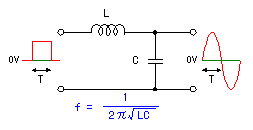As for the square wave signal which was made with the oscillator, the maximum voltage is 5Vp-p(Low:0V, High:+5V). current signal by the resonance circuit which used the coil and the capacitor. The positive and the negatively changing alternating current signal are composed of the resonance circuit. The resonant frequency becomes the frequency to take out. The resonant frequency is calculated in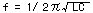. When calculating as L = 18 µH, C = 330 pF, the resonant frequency becomes about 2 MHz.At the circuit which I made, the resonant frequency was 2,140 KHz.The voltage amplification rectification circuit This is the circuit which gets about twice of DC voltages from the inputted alternating voltage.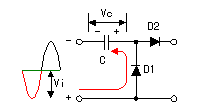In the period with the negative voltage of the input alternating current signal, the electric charge is stored up to the capacitor (C) through D1. With this, the voltage of the both edges of the capacitor becomes Vc.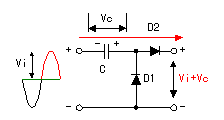Next, in the period with the positive voltage with the input alternating voltage, the input voltage (Vi) and the voltage (Vc) of the capacitor are gained and the voltage of Vi+Vc is output. The value of Vi and Vc are changed by the frequency of the input alternating current or the electric current which flows through the load. The circuit this time works in the high frequency switching. Because it is, as the diode, the shottky barrier diode with the short recovery time must be used.The ripple filter circuit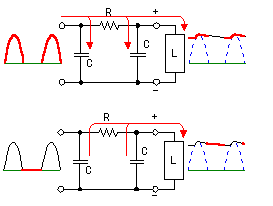As for the DC voltage which is output from the voltage amplification rectification circuit, the half wave was rectified. So, the ripple is contained in the output. The ripple filter circuit is used to make this ripple little. The circuit this time is using the resistor instead of the coil. Therefore, the output voltage falls at more when the output current increases.